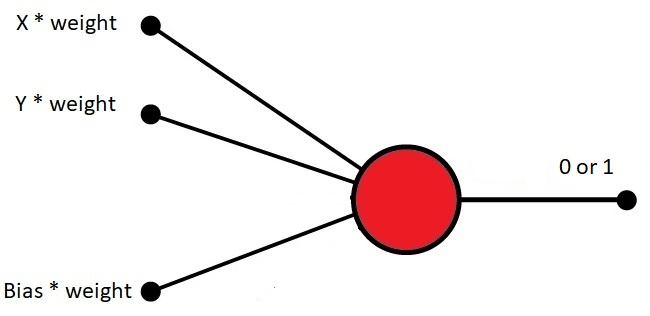# Pattern Recognition

Neural Networks are used in applications like Facial Recognition.

These applications use Pattern Recognition.

This type of Classification can be done with a Perceptron.

Perceptrons can be used to classify data into two parts.

Perceptrons are also known as a Linear Binary Classifiers.

## Pattern Classification

Imagine a strait line (a linear graph) in a space with scattered x y points.

How can you classify the points over and under the line?

A perceptron can be trained to recognize the points over the line, without knowing the formula for the line.## How to Program a Perceptron

To program a perceptron, we can use a simple JavaScript program that will:

1. Create a simple plotter
2. Create 500 random x y points
3. Display the x y points
4. Create a line function: f(x)
5. Display the line

## Create a Simple Plotter

Creating a simple plotter object is described in the AI Canvas Chapter.

### Example

const plotter = new XYPlotter("myCanvas");
plotter.transformXY();

const xMax = plotter.xMax;
const yMax = plotter.yMax;
const xMin = plotter.xMin;
const yMin = plotter.yMin;

## Create Random X Y Points

Create as many xy points as wanted.

Let the x values be random (between 0 and maximum).

Let the y values be random (between 0 and maximum).

Display the points in the plotter:

### Example

const numPoints = 500;
const xPoints = [];
const yPoints = [];
for (let i = 0; i < numPoints; i++) {
xPoints[i] = Math.random() * xMax;
yPoints[i] = Math.random() * yMax;
}

Try it Yourself »

## Create a Line Function

Display the line in the plotter:

### Example

function f(x) {
return x * 1.2 + 50;
}

Try it Yourself »

Compute the correct answers based on the line function:

y = x * 1.2 + 50.

The desired answer is 1 if y is over the line and 0 if y is under the line.

Store the desired answers in an array (desired[]).

### Example

let desired = [];
for (let i = 0; i < numPoints; i++) {
desired[i] = 0;
if (yPoints[i] > f(xPoints[i])) {desired[i] = 1;}
}

For each point, if desired[i] = 1 display a black point, else display a blue point.

### Example

for (let i = 0; i < numPoints; i++) {
let color = "blue";
if (desired[i]) color = "black";
plotter.plotPoint(xPoints[i], yPoints[i], color);
}

Try it Yourself »

## How to Train a Perceptron

In the next chapter, you will learn how to use the correct answers to:

Train a perceptron to predict the output values of unknown input values.

W3Schools is optimized for learning and training. Examples might be simplified to improve reading and learning. Tutorials, references, and examples are constantly reviewed to avoid errors, but we cannot warrant full correctness of all content. While using W3Schools, you agree to have read and accepted our terms of use, cookie and privacy policy.